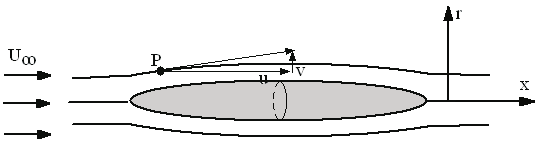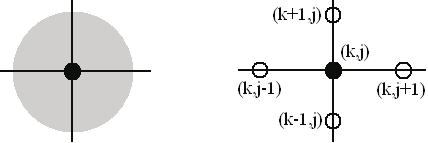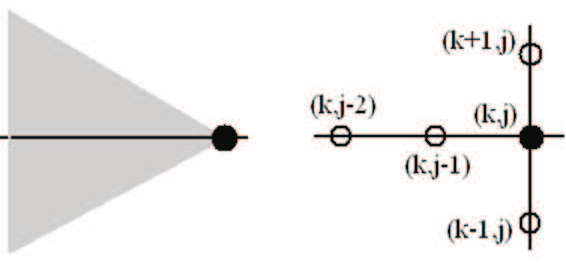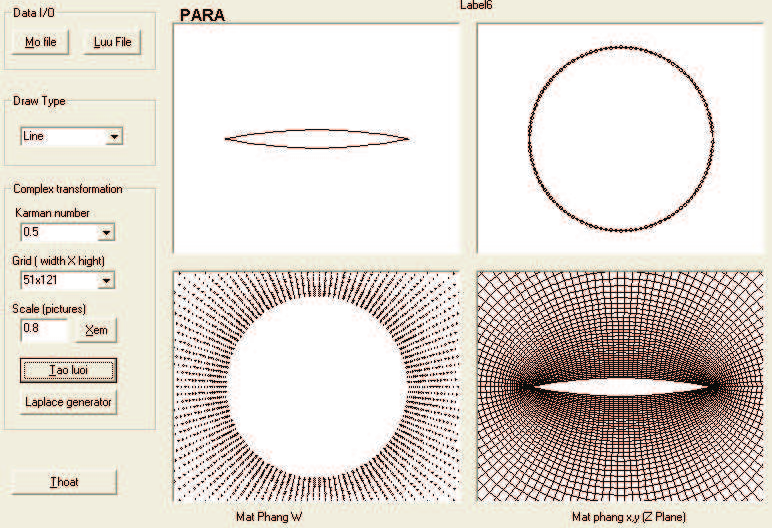Vietnam Journal of Mechanics, VAST, Vol.32, No.1 (2010), pp. 27–36
STUDY OF TRANSONIC EFFECT OF FLOWS
AROUND SLENDER BODIES OF REVOLUTION
AND PLANE PROFILES
Hoang Thi Bich Ngoc
Hanoi University of Technology
Abstract. Transonic effectwithpresence of local supersonic terminated by shock wave is
not wished. In spite of wishes or not, the appearance of shock waves is not avoided. Strong
shock waves cause losses, even a grand decrease of aerodynamic quality in fluid-solid
interaction. However, under certain conditions, transonic effect increases the drag and
at the same time increases the lift leading the amelioration of aerodynamic quality. This
work uses numerical methods and established programs for solving differential equations
of the axisymmetric flow and the plane flow to study the influence of transonic effects
for the two cases.
1.
INTRODUCTION
Shock wave is a special phenomenon for supersonic flows. Transonic flows include
supersonic regions, so the shock wave is a phenomenon usually attached to transonic
flows. Properties of shock wave depend on the geometry and free Mach number. For
the same main section, transonic effects are very much different between plane flow and
flow of revolution. With the hypothesis of potential flow, the calculation of flows around
slender bodies of revolution is carried out by solving small disturbance potential equations.
Numerical results of the established program are compared with published experimental
and numerical results.
For flows around plane profiles, transonic effect attached shock wave much stronger
than transoniceffect inaxisymmetricflowsunder the sameconditions. Therefore, the small
disturbance method isnot adequatefor planeflows.In thiscase, solving full potential equa-
tions is used. Numerical results of established program are compared with experimental
results. This work uses also the Fluent software to calculate transonic flows. Numerical
results calculating from the established program and results of Fluent are compared each
other.
2.
NUMERICAL METHODS
2.1.
Transonic flow around slender bodies of revolution
The problem of slender axisymmetric transonic flow is solved by using small distur-
bance method with aerodynamic bodies having maximum thickness ratios less than 10%2
a
∂x ∂r
2 2 2 2
∂ ϕ ∂ ϕ ∂ ϕ a
1
ϕ
x
2 2
28
Hoang Thi Bich Ngoc
and without incidence. The full potential equation for the axisymmetric flow is written in
cylindrical coordinate system :
a2 u2Φxx + a2 v2Φrr + r Φr 2uvΦxr = 0
(1)
where Φ is velocity potential function, u = Φx; v = Φr; a is velocity of sound.
Fig. 1. Axisymmetric flow
Conditions of small disturbance for velocities are written as: u = U + u0 ; v =
0 +v0 = v0
(u0 and v0 are disturbance velocities in the directions x and r)
Φ = x.U+ϕ
(2)
where ϕ isdisturbance velocitypotential function: ∂ϕ = u0 ; ∂ϕ = v0 The small disturbance
potential equations is written as follows:
a2 u2 ∂x2 2uv∂x∂r + a2 v2 ∂r2 + r ϕr = 0
(3)
Using the Mach number M, the equation (4) becomes:
  
1 M(γ +1)MUϕxx + r (r)r = 0
(4)
where γ is ratio of specific heats at constant pressure and constant volume γ = cp/cv.
The equation (4) with the boundary conditions of slip on the profile is solved by
a method of finite differences. For flow in subsonic regions, the partial differential equa-
tion (4) is under the elliptic form, and in supersonic regions, this equation takes in the
hypersonic form .
The equation (4) allows transforming from the elliptic form into the hyperbolic form
and vice versa by using the following expression:
λ = β2 M2 (γ + 1)ϕx;
β2 = 1 M2
(5)
2.2.
Transonic flow around plane profiles
Disturbances of plane flow are very much stronger than those of axisymmetric flow,
and thus, the small disturbance method is conveniently applied only to plane profiles
having thickness less than 6%. Transonic flow problem for a plane profile is thus treated" # " #
a + a − −2 = 0
Study of transonic effect of flows around slender bodies of revolution and plane profiles
29
by solving the full potential equation. For two-dimensional flow, the full potential equation
is written in Cartesian coordinate system :
2 Φ2 2Φ 2 Φ2 2Φ ΦΦ 2Φ
∂x ∂x2 ∂y ∂y2 ∂x ∂y ∂x∂y
(6)
In the case of symmetric geometry and cinematic, the boundary conditions for the
equation (6) are the condition of slip on profile and the disturbance is negligible at the
infinity. The equation (6) is solved on a grid of finite differences. A center diagram is used
for an elliptic region (Fig. 2), and two-step diagram for a hyperbolic region (Fig. 3).
Fig. 2. Difference in elliptic region
Fig. 3. Difference in hyperbolic region
Being different from the small disturbance method, the full potential equation prob-
lem requires a grid much more complex. In this work, the grid is generated by using two
methods: iterating the Laplace’s equation and mapping transformation.
Fig. 4. Process of grid generation for parabolic-arc profile L/D=10 - results from established
program using mapping transformation